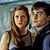# Only People Who Majored In Math Can Pass This Fractions Test

## Fractions are easy right? Wrong. Fractions can be super complicated! Can you determine the decimal number for each of these fractions and vice versa? Let's see!Created by Carly Wallace
On Feb 13, 2023

Get out your protractor and calculator! These questions will test your knowledge of fractions and decimal numbers! It's not aways easy to determine fractional numbers. Let's see if you can pass this difficult fractions quiz!

1 / 10

1/2
What is the decimal number for this fraction?

2 / 10

0.35
What is the fraction for this decimal number? Can you guess?

3 / 10

0.55
Now let's see, what is the fraction of this decimal number?

4 / 10

3/4

This one's easy what is the decimal number of this fraction?

5 / 10

12/8

Ok, what is the decimal number of this fraction?

6 / 10

0.88

What is the fraction of this decimal number?

7 / 10

0.12

This one's a bit harder, what is the fraction of this decimal number?

8 / 10

4/5

Hmm, what is the decimal number of this fraction?

9 / 10

9/10

What is the decimal number of this fraction?

10 / 10

7/5
Ohh, this one's a little challenging...What is the decimal number of this fraction?

10
Questions left

### What math tool can you not live without?

Calculating results
These are 10 of the World CRAZIEST Ice Cream Flavors
Created by Tal Garner
On Nov 18, 2021